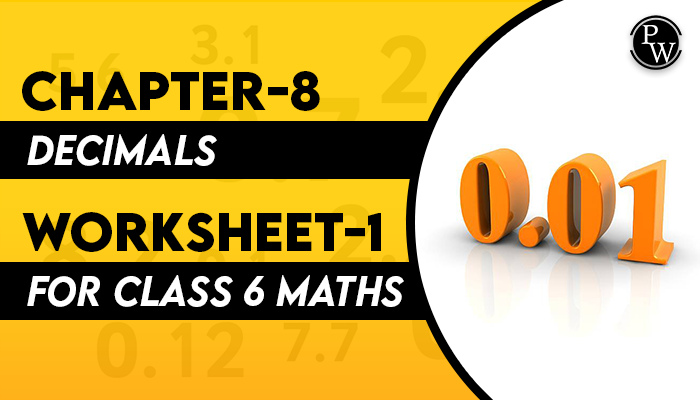# Chapter-8 Decimals Worksheet-1 for class 6 maths

## Decimals

In Algebra, decimals are one of the kinds of numbers, that have a whole number and the fractional part separated with a decimal point. The dot present between the whole number and fractions part is known as the decimal point. For example, 44.5 is a decimal number.

Here, 44 is a whole number part and 5 is the fractional part. “.” is the decimal point.

### The necessary properties of decimal numbers under multiplication and division operations are as follows:

1. If any of the 2 decimal numbers are multiplied in any order, the product remains the same.
2. If a whole number and a decimal number are multiplied in any order, the product remains the same.
3. If a decimal fraction is multiplied by 1, the product is the decimal fraction itself.
4. If a decimal fraction is multiplied by zero, the product is 0.
5. If a decimal number is divided by 1, the quotient is the decimal number.
6. If a decimal number is divided by the same number, the quotient is 1.
7. If 0 is divided by any decimal, the quotient remains 0.
8. The division of a decimal number by 0 isn't possible, as the reciprocal of 0 doesn't exist.The Academic team of Physics Wallah prepared worksheets and questions for chapter Decimals for all students who are in class 6. Physics Wallah questions for class 6 maths chapter Decimals consist of carefully selected numerical for class 6 maths and consist of step by step solutions of all questions with answers. Download Worksheet - 1 of Class 6 Maths Chapter Decimals and start solving the questions for reference use the solutions.

Before solving the worksheet of Physics Wallah it is highly recommended for reading the theory of the chapter and solving the questions given in your textbook. Do follow NCERT Solutions for class 6 Maths prepared by Physics Wallah.

### Solved Questions

Q1. Express 360 mm as metre.
1. 36 m
2. 3.06 m
3. 3.6 m
4. 0.36 m

Ans. Correct Option is 4

1 m = 1000 mm, so,

360/1000 = 0.36 m.

Q2. 5.4 lie in between which two whole numbers?

1. 0 – 1
2. 4 – 5
3. 5 – 6
4. 10 – 11

Ans. The Correct option is 3

5.4 lies in between 5 – 6, as it is less than 6 but more than 5.

Q3. The length of Harsh’s book is 8 cm 4 mm. What will be the length in cm?
1. 8.4 cm
2. 8.6 cm
3. 0.86 cm
4. None of them 7.

Ans. The Correct option is 1

1 cm = 10 mm,

So, 4 mm = 0.4 cm.

Hence, 8 + 0.4 = 8.4 cm.

Q4. Which of the following is greater? 1.008 or 1.800
1. 1.008
2. 1.800
3. Both are equal
4. None of them

Ans. The Correct Option is 2

1.8 > 1.008.

Q5. How many km in 2008 m?
1. 2.008 km
2. 20.08 km
3. 200.8 km
4. 0.2008 km

Ans. The Correct option is 1

1 km = 1000 m,

So, 2008 / 1000 = 2.008.

Q6. Which of the following statements is true?

1. Every decimal cannot be written as a fraction.
2. All decimals cannot be represented on the number line.
3. Every fraction with denominator 10 can be written in decimal notation and vice versa.
4. All of the above statements are true

Ans. The Correct answer is 3

Using the decimal system, every number can be written in the form of a fraction of it has either the numerator or denominator as 10 or any of its multiple.

### Class 6 Maths Chapter - 8 Decimals download your free pdf copy from the below-mentioned link.